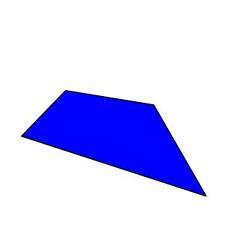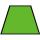# Trapezium

The area of trapezium is 35 cm2. Find its altitude if the bases are 6cm and 8 cm.

Correct result:

h =  5 cm

#### Solution:We would be pleased if you find an error in the word problem, spelling mistakes, or inaccuracies and send it to us. Thank you!#### You need to know the following knowledge to solve this word math problem:

We encourage you to watch this tutorial video on this math problem:

## Next similar math problems:

• TrapeziumThe area of trapezium is 35cm2 find its altitude if the bases are 6cm and 8cm.
• Trapezium basesFind the trapezium height if a = 8 cm and c = 4 cm if its content 21 square centimeters.
• Isosceles trapezoidFind the area of an isosceles trapezoid, if the bases are 12 cm and 20 cm, the length of the arm is 16 cm
• Area of iso-trapFind the area of an isosceles trapezoid if the lengths of its bases are 16 cm and 30 cm, and the diagonals are perpendicular to each other.
• IS trapezoidIsosceles trapezoid arm measured 35 cm. Height is 30 cm and middle segment is 65 cm. Determine length of its bases.
• R TrapeziumRectangular trapezium has bases 12 and 5 and area 84 cm2. What is its perimeter?
• ISO trapezoid v2bases of Isosceles trapezoid measured 20 cm and 4 cm and its perimeter is 55 cm. What is the are of a trapezoid?The quadrangular prism has a volume of 648 cm3. Trapezoid which is its base has the dimensions bases: a = 10 cm, c = 5 and height v = 6 cm. What is the height of the prism?
• Ratio in trapeziumThe height v and the base a, c in the trapezoid ABCD are in the ratio 1: 6: 3, its content S = 324 square cm. Peak angle B = 35 degrees. Determine the perimeter of the trapezoid
• The basesThe bases of the isosceles trapezoid ABCD have lengths of 10 cm and 6 cm. Its arms form an angle α = 50˚ with a longer base. Calculate the circumference and content of the ABCD trapezoid.
• Rectangular trapeziumCalculate the perimeter of a rectangular trapezium when its content area is 576 cm2 and sice a (base) is 30 cm, height 24 cm.
• Trapezoid - intersection of diagonalsIn the ABCD trapezoid is AB = 8 cm long, trapezium height 6 cm, and distance of diagonals intersection from AB is 4 cm. Calculate the trapezoid area.
• TrapezoidArea of trapezoid is 135 cm2. Sides a, c and height h are in a ratio 6:4:3. How long are a,c and h? Make calculation...
• Trapezoid MO-5-Z8Trapezoid KLMN has bases 12 and 4 cm long. The area of triangle KMN is 9 cm2. What is the area of the trapezoid KLMN?
• Uboid volumeCalculate the cuboid volume if the walls are 30cm², 35cm², 42cm²
• Isosceles trapezoidCalculate the content of an isosceles trapezoid whose bases are at ratio 5:3, the arm is 6cm long and it is 4cm high.
• TrapezoidHow long are the trapezoid bases with area 24 cm2 and height 3 cm. One base is 3 times longer than the shorter.